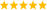# LabVIEW

cancel
Showing results for
Did you mean:

Highlighted

## Find two unknowns in equation from 38301 dataset

I am using a thermopile whose dataset is available around 38301 values. This is purely mathematical query. Need to find two unknowns in below equation:

Vtp = K( Tobj^(4-n) - Ta^(4-n) )

Company says K & n are empirically determined value & this they wont tell.

Vtp is in volts
Tobj & Ta are in kelvins

Again this is purely mathematical query, just to see if it can be find out.

From these 38301 dataset values, we need to find best fit values of K & n, so that in final calculations when Tobj is to be calculated from known values of Vtp,Ta,K & n, this has minimum error.

Message 1 of 4
(148 Views)
Highlighted

## Re: Find two unknowns in equation from 38301 dataset

After all our discussions last months, this seems trivial. What have you tried?

You can fit for the best parameters using the current formula, but to calculate Tobj, you need to solve for Tobj, of course. Doesn't look hard.

The column labels in your data file make no sense. Tntc does not show in your formula, and why are they to the fourth power. (Tobj^4, Tntc^4). if these are really the values, shouldn't the units be Kelvin^4? I can guarantee that you don't need 20+ significant digits for VTP. What a waste of bytes! How many bits does the digitizer actually have?LabVIEW Champion. It all comes together inGCentralWhat does "Engineering Redefined" mean??
Message 2 of 4
(122 Views)
Highlighted

## Re: Find two unknowns in equation from 38301 dataset

1. Sorry this is NTC and Tobj tempearture in kelvin.  This ^4 is wrong mentioned. This is typo. Please ignore

2. My actual area of interest was 30 to 47C obj & Tntc=0 to 50, I have been able to make equation with +-0.07C in this region , my practical purpose is solved.

3. This is just mathematical thing, i want to see if it can fit mathematically also thats it.

Message 3 of 4
(104 Views)
Highlighted

## Re: Find two unknowns in equation from 38301 dataset

Hi Vindhy,

@Vindhyachal.Takniki wrote:

2. My actual area of interest was 30 to 47C obj & Tntc=0 to 50

3. This is just mathematical thing, i want to see if it can fit mathematically also thats it.

Here you can see the result after applying "mathematically fit":I used the range from -50°C…100°C…

Best regards,
GerdWusing LV2011SP1 + LV2017 (+LV2020 sometimes) on Win10+cRIO
Message 4 of 4
(43 Views)